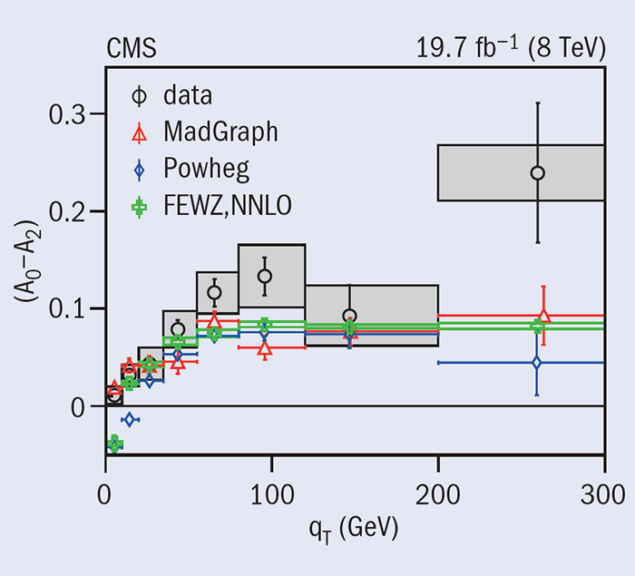# CMS digs deeply into lepton-pair production

27 April 2015The measured difference of the angular coefficients, A0–A2, as a function of the transverse momentum, qT, confirms the anticipated deviation from the Lam–Tung relation (A0 = A2).

Lepton pairs produced in proton–proton collisions at the LHC provide a clear signal that is easy to identify in the detector. The production is dominated by the Drell–Yan process, in which an intermediate Z/γ* boson is produced by the incoming partons. The measurements of the Drell–Yan production cross-section as a function of the mass of the intermediate boson, its rapidity (corresponding to the scattering angle) and its transverse momentum allow sensitive tests of QCD, the theory of the strong interaction. Recently, the CMS collaboration published two new measurements that provide a comprehensive view of the production of dimuons, a pair of oppositely charged muons, via the decay of Z bosons at a collision energy of 8 TeV at the LHC.

The parton structure of the proton and its evolution, governed by the dynamics of the strong interaction, can be scrutinized over a large range of phase space. By comparing the measurements to calculations that employ different parton distribution functions (PDFs) and different theoretical models for the dynamics, the PDFs and their uncertainty can be improved. These studies are also important for investigating other physics processes, for example searches for new resonances decaying into dileptons in models beyond the Standard Model.

In the CMS analysis, dimuon production in the vicinity of the Z-boson peak was parameterized doubly differentially as functions of the transverse momentum (qT) and the rapidity (y) of the Z boson. The analysis used the data sample of proton–proton collisions at a centre-of-mass energy of 8 TeV, amounting to an integrated luminosity of 19.7 fb–1. The measurement probes the production of Z bosons up to high transverse momenta of qT > 100 GeV, a kinematic regime in which the production is dominated by gluon–quark fusion. Therefore, the measurement is sensitive to the gluon PDF in a kinematic regime that is important for Higgs-boson production via gluon fusion. In the future, Z-boson production can also be used to constrain the gluon PDF and provide information complementary to other processes employed, such as direct photon production. The data are well reproduced within uncertainties by the next-to-next-to-leading-order predictions computed with the FEWZ simulation code. The MADGRAPH and POWHEG predictions deviate from data up to 20% at high-z transverse momentum.

CMS has measured the five major angular coefficients A0 to A4 as a function of qT and y

The angular distribution of the final-state leptons in Drell–Yan production is determined by the vector and axial-vector coupling structure of the Standard Model Lagrangian, and by the relative contributions of the quark–antiquark annihilation and quark–gluon Compton processes. In the presence of higher-order QCD corrections, the general structure of the lepton angular distribution in the boson rest-frame is given by a formula that contains a set of angular coefficients.

Using the 8 TeV data, CMS has measured the five major angular coefficients A0 to A4 as a function of qT and y. None of the theoretical models tested describe all of the coefficients satisfactorily. The coefficients A0 and A2 measured by CMS in proton–proton collisions at the LHC are larger than those measured in proton–antiproton collisions at Fermilab’s Tevatron at a lower centre-of-mass energy. This is expected, owing to the significant contribution of the quark–gluon process in proton–proton collisions at the LHC. In addition, as the figure shows, the analysis confirmed for the first time the anticipated deviation from the Lam–Tung relation, A0 = A2 (Lam and Tung 1979). This deviation is expected in QCD calculations beyond the leading order. The measurement by CMS shows that A0 > A2, especially for high qT. Nonzero values were also measured for A1 and A3.

The comprehensive study of the Z-boson production mechanism presented in these two recently published CMS papers lays the foundation for future high-precision measurements, such as the measurement of the mass of the W boson and the electroweak mixing angle.